# 红黑树的性质

1. 节点是红色或黑色。
2. 根是黑色。
3. 所有叶子都是黑色（叶子是NIL节点）。
4. 每个红色节点必须有两个黑色的子节点。（从每个叶子到根的所有路径上不能有两个连续的红色节点。）
5. 从任一节点到其每个叶子的所有简单路径都包含相同数目的黑色节点。
6. 红链接均为左链接（这种树被称为：Left Lean RedBlack Tree）

# 插入节点操作

#### 2-3树的表示

1.单个2-节点插入：直接插入即可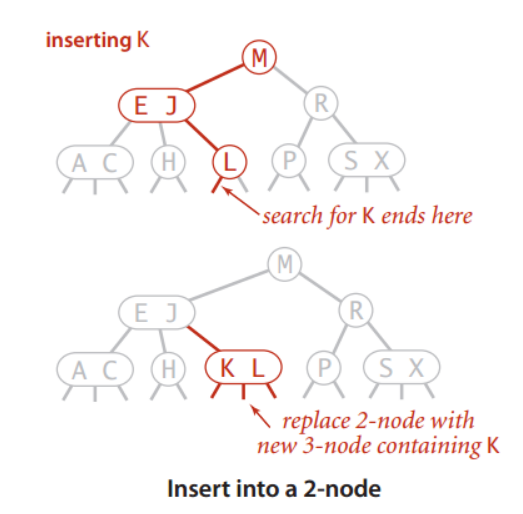2.单个3-节点插入：会生成一个临时的4-节点。需要将中间的节点分解为3个2-节点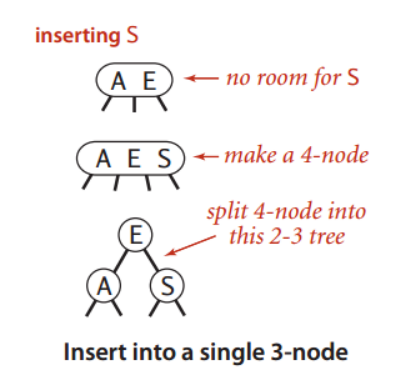3.父节点为2-节点的3-节点插入：生成临时的4-节点，如果需要沿着递归插入的路径向上分解为三个2-节点。父节点变成一个3-节点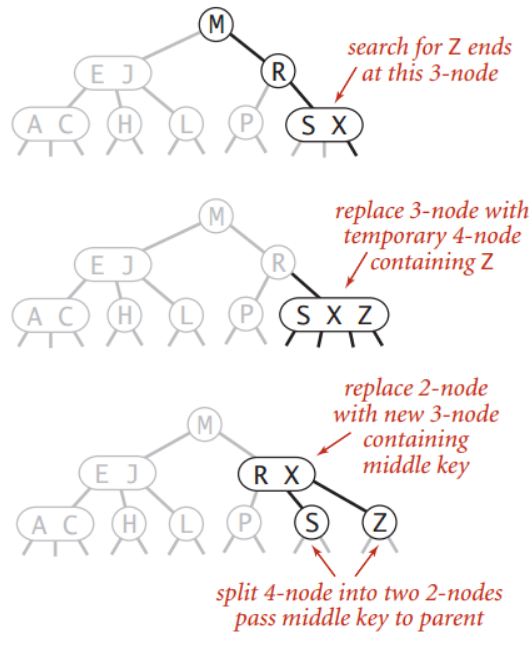4.父节点为3-节点的3-节点插入：也是需要分解，但是只要遇到父节点是2-节点或者是根节点就停止。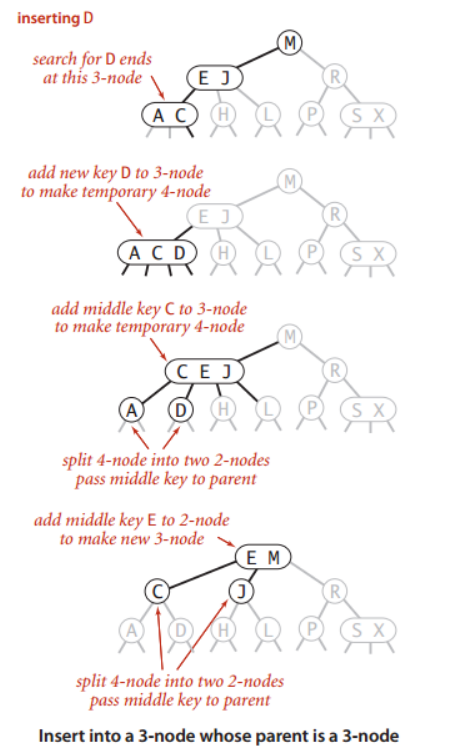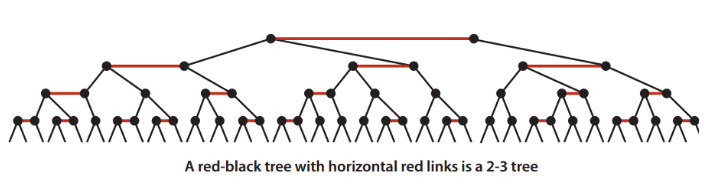# 红黑树的插入操作

《算法（第四版）》的C#实现。红黑树的定义：

// 符号表的抽象类
public abstract class SymbolTablet<Key, Value>
where Key : IComparable
{
public abstract void Put(Key key, Value value);
public abstract Value Get(Key key);
public abstract void Delete(Key key);
public abstract bool Contains(Key key);
public abstract bool IsEmpty();
public abstract int Size();
public abstract Key Min();
public abstract Key Max();

public abstract int Rank(Key key);
//排名为k的键
public abstract Key Select(int k);
//删除最小的键
public abstract void DeleteMin();
public abstract void DeleteMax();

}
//红黑树的定义
class RBTree<Key, Value>: SymbolTablet<Key, Value>
where Key:IComparable
{
//定义颜色 readonly == final in Java
private static readonly bool RED = true;
private static readonly bool BLACK = false;
//构造函数
public RBTree()
{
root = null;
}
//根节点
private Node root;
//定义节点
internal class Node
{
internal Key key;
internal Value val;
internal Node left, right;//左右的儿子
internal bool color;
internal int N;//这棵树的总子节点数量
//构造函数
internal Node(Key key, Value val, int N, bool color)
{
this.key = key;
this.val = val;
this.N = N;
this.color = color;
}
//打印数据
public override string ToString()
{
return key.ToString() + ":" + val.ToString();
}
}
....
}

# 红黑树的通用操作

//修复操作
//左旋转h节点
private Node rotateLeft(Node h)
{
Node x = h.right;
h.right = x.left;
x.left = h;
x.color = h.color;
h.color = RED;
x.N = h.N;
h.N = 1 + Size(h.left) + Size(h.right);
return x;
}
//右旋转
private Node rotateRight(Node h)
{
Node x = h.left;
h.left = x.right;
x.right = h;
x.color = h.color;
h.color = RED;
x.N = h.N;
h.N = Size(h.left) + Size(h.right) + 1;
return x;
}
//反转颜色
private void flipColors(Node h)
{
//转换颜色
h.color = !h.color;
h.left.color = !h.left.color;

h.right.color = !h.right.color;

}

### 调整方面的操作

1.由于是局部的调整，不需要考虑h节点本身是什么颜色，这需要程序根据实际的情况进行调用。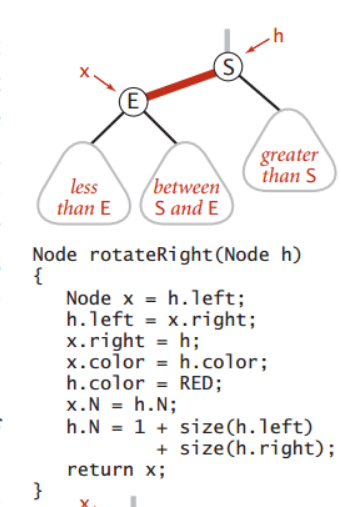2.同理，左旋转修复。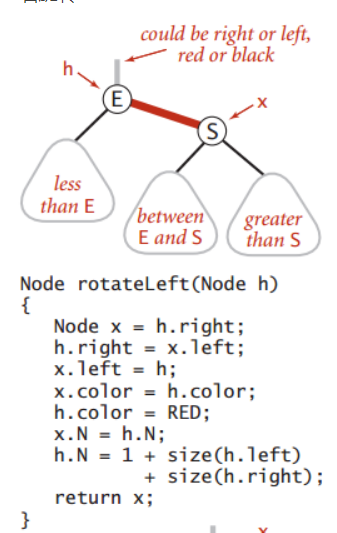### 插入的情况

1.向局部2-节点插入：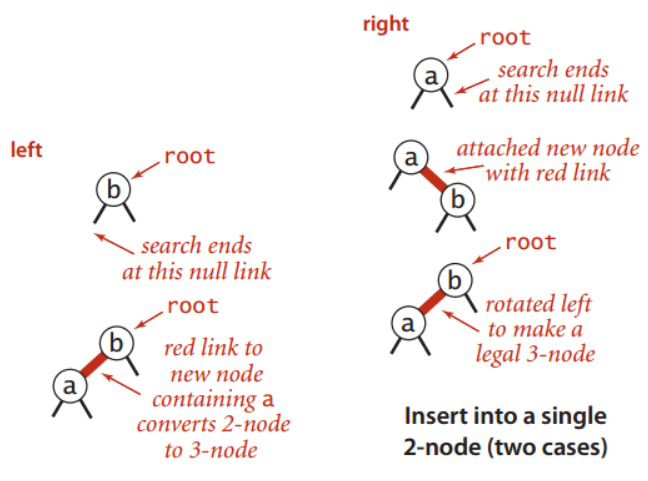2.向树底的2-节点插入：如果插入在父节点的有子节点，需要进行左旋转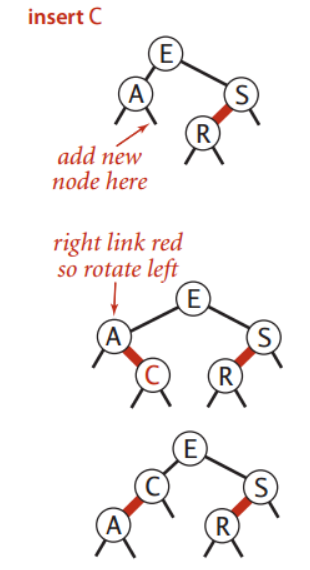2.向局部的3-节点插入：

• 小于最小的原有节点：插入到左边的红节点之下，产生两个连续的红节点，在中间节点进行右旋转。变为根节点为黑节点且拥有两个红节点。但是右子树中出现红节点，最后进行flipColor处理。将红节点向树根传递。
• 介于原有节点之间：即根节点h的h.lefth.left.right为红节点，所以以h.left 为轴左旋，然后回到了第一种情况。
• 大于最大的原有节点：插入为右红节点，类似于第一种情况的加粗描述的情况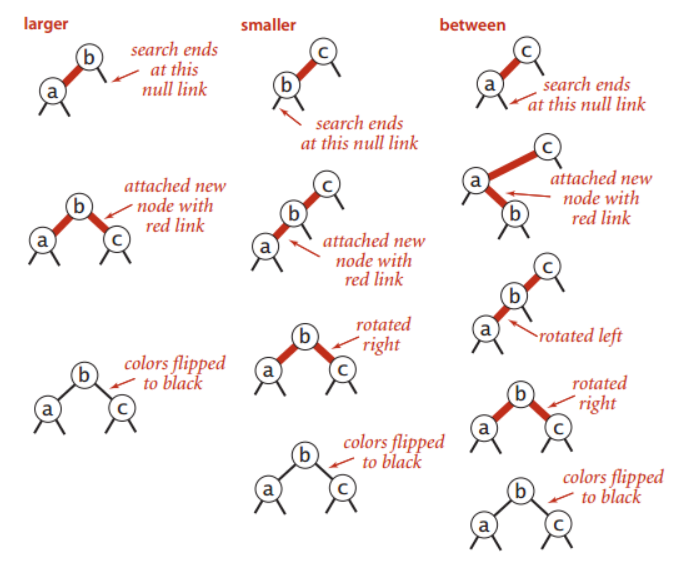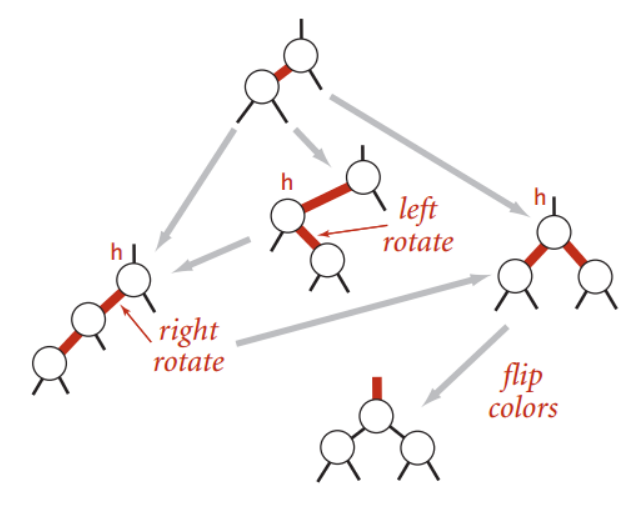C#实现：

//插入节点的接口和实现
//插入节点
private Node Put(Node h, Key key, Value val)
{
if (h == null)
return new Node(key, val, 1, RED);
int cmp = key.CompareTo(h.key);
if (cmp < 0)
h.left = Put(h.left, key, val);
else if (cmp > 0)
h.right = Put(h.right, key, val);
else
h.val = val;
//调整
if (IsRed(h.right) && !IsRed(h.left))
h = rotateLeft(h);//按照红黑树的定义，红色的节点必须在左儿子处
//是否左边连续两个红色
if (IsRed(h.left) && IsRed(h.left.left))
h = rotateRight(h);
//反转颜色
if (IsRed(h.left) && IsRed(h.right))
flipColors(h);
h.N = Size(h.left) + Size(h.right) + 1;
return h;
}

public override void Put(Key key, Value value)
{
root = Put(root, key, value);
}

# 删除操作

### 2-3-4树

• 4-节点分解为三个2-节点，父2节点与两个孩子使用红色连接。
• 向下的过程中分解所有4-节点
• 向上的过程中，将4-节点右旋配平

### 删除最小元素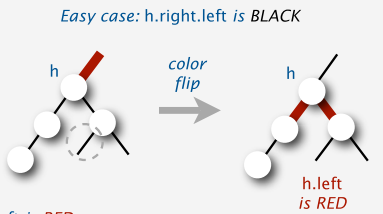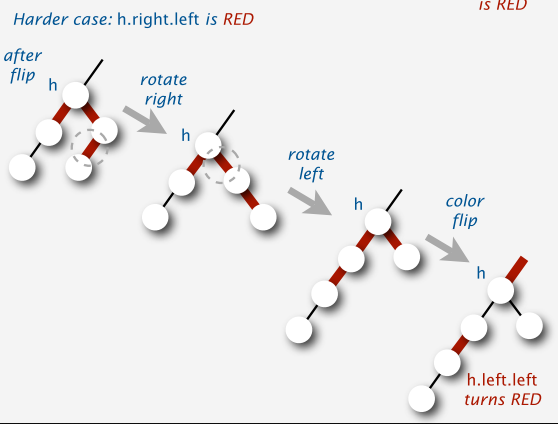C#的实现：

//旋转修复
//删除的自底向上的修复
private Node Balance(Node h)
{
//调整
if (IsRed(h.right))
h = rotateLeft(h);//按照LLRB红黑树的定义，红色的节点必须在左儿子处
if (IsRed(h.right) && !IsRed(h.left))
h = rotateLeft(h);//按照红黑树的定义，红色的节点必须在左儿子处
//是否左边连续两个红色
if (IsRed(h.left) && IsRed(h.left.left))
h = rotateRight(h);
//反转颜色
if (IsRed(h.left) && IsRed(h.right))
flipColors(h);
h.N = Size(h.left) + Size(h.right) + 1;
return h;
}
//左旋转h节点
private Node rotateLeft(Node h)
{
Node x = h.right;
h.right = x.left;
x.left = h;
x.color = h.color;
h.color = RED;
x.N = h.N;
h.N = 1 + Size(h.left) + Size(h.right);
return x;
}
//右旋转
private Node rotateRight(Node h)
{
Node x = h.left;
h.left = x.right;
x.right = h;
x.color = h.color;
h.color = RED;
x.N = h.N;
h.N = Size(h.left) + Size(h.right) + 1;
return x;
}
//删除最小的节点
private Node moveRedLeft(Node h)
{
//假设节点h为红色，h.left 和h.left.left 是黑色的
//将h.left 或者是h.left 的子节点之一变红。
flipColors(h);//我们需要得到最小的节点是红孩子。按照性质红节点的两个孩子是黑色的。需要反色处理
if(IsRed(h.right.left))//处理违反性质的情况：红孩子的节点不是黑节点
{
h.right = rotateRight(h.right);//都变成连续的右红节点。
h = rotateLeft(h);//右子树右旋了。红节点到了右边不符合规定。然后h左旋，变成了一个四节点的树
//可以给左子树形成一条红-黑-红的路线
//flipColors(h);//上一条语句执行后，违反了性质（旋转后红色的根节点的右孩子还是红色），需要重新设置颜色。
//再次反色形成黑-红-红。成为3-节点
flipColors(h);
}
return h;
}
private Node DeleteMin(Node h)
{
if (h.left == null)
return null;
if (!IsRed(h.left) && !IsRed(h.left.left))//处理的情况：不是左红孩子。要从右边借一个红孩子过来
h = moveRedLeft(h);//给左子树添加孩子
h.left = DeleteMin(h.left);
return Balance(h);

}
public override void DeleteMin()
{
if (!IsRed(root.left) && !IsRed(root.right))
root.color = RED;//根节点设置为红色
root = DeleteMin(root);
if (!IsEmpty())
root.color = BLACK;
}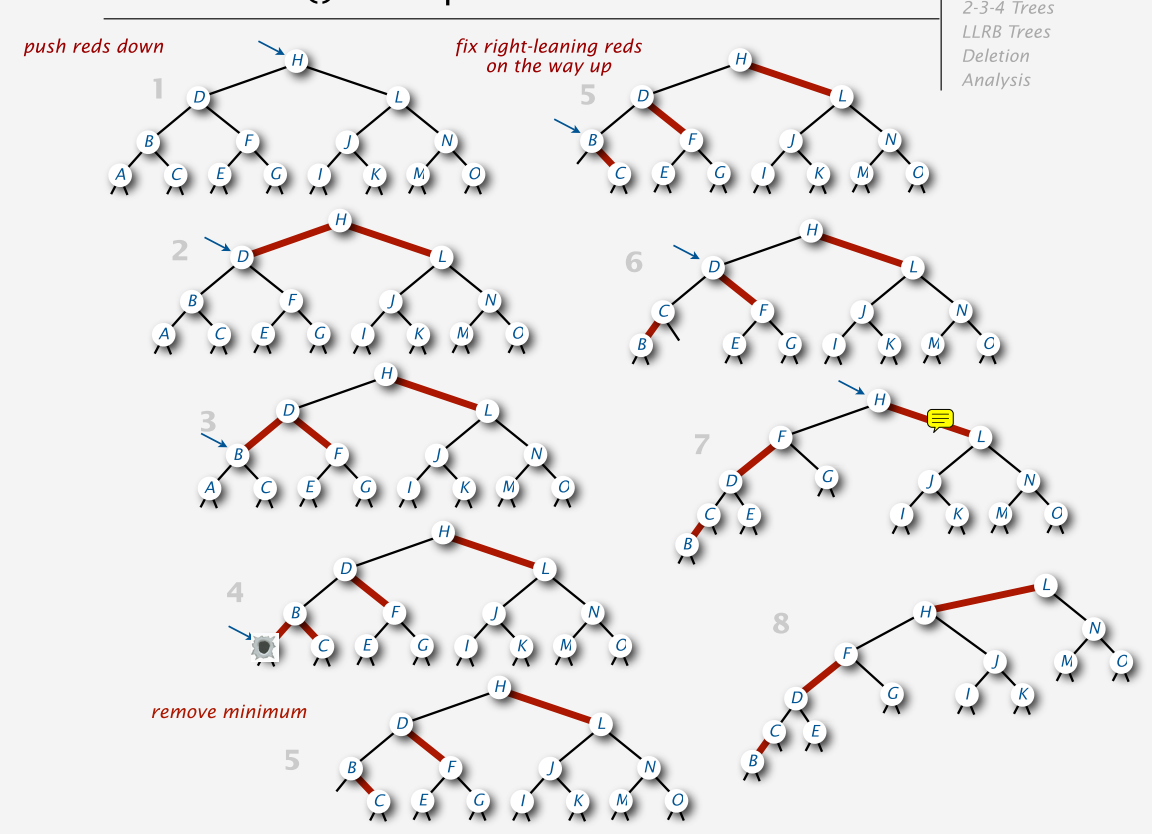### 删除最大元素

1.假设最右边的是3-节点。由于LLRB红黑树的红节点在最左侧，所以用一次右旋转就可以形成一个红节点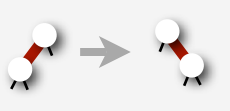2.如果左孙子是黑色的，那么可以在根进行颜色反转就可以了。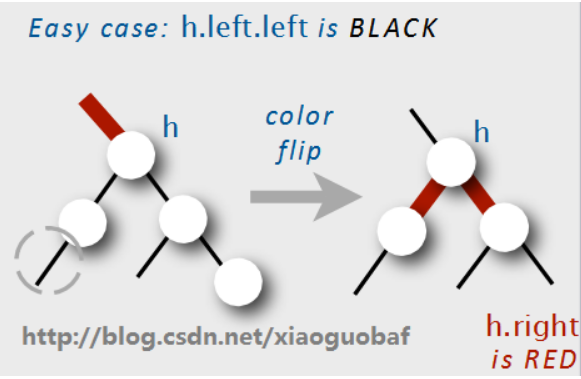3.如果左孩子的左孩子是红色的，那么就可以以h.left为轴进行右旋转。这样红节点向上了，但是这就不能维持红黑树的定义（其实可以在最后使用Balance进行修复）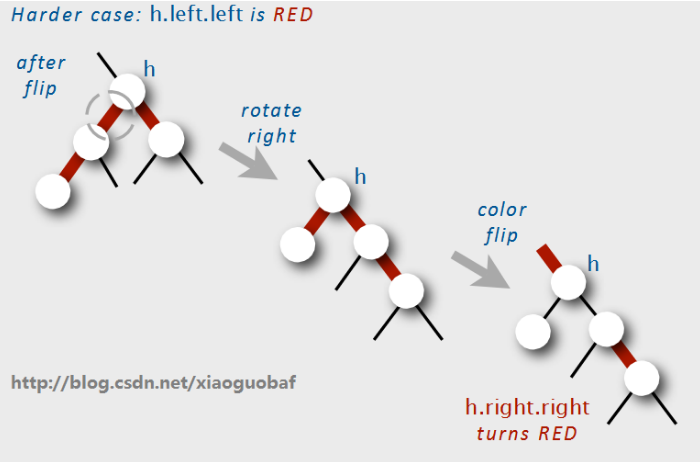C#的实现：

//---删除最大的节点
private Node moveRedRight(Node h)
{
//假设节点h为红色，h.right 和h.right.left 是黑色
//将h.right 或者h.right的子节点之一变红
flipColors(h);//红节点向下
if (IsRed(h.left.left))//左孙子是一个红节点，我需要借一个过来到右边来。
{
h = rotateRight(h);//右旋转添加两个连续个红节点
flipColors(h);//右子树为红-原左子树的节点的颜色-红。再次反色就可以向下传递
}

else
{
//h = rotateRight(h);//右旋转添加两个连续个红节点
//flipColors(h);
//左边出现的红-红可以被修复
}

return h;
}
private Node DeleteMax(Node h)
{
if (IsRed(h.left))//左孩子是红节点，说明这个节点是3-节点。由于红节点在左侧，所以右旋即可/
h = rotateRight(h);
if (h.right == null)
{
Console.WriteLine("删除了节点：{0}", h.ToString());
return null;
}
if (!IsRed(h.right) && !IsRed(h.right.left))
h = moveRedRight(h);//给右子树添加一个红节点
h.right = DeleteMax(h.right);
return Balance(h);
}
//删除最大节点的接口
public override void DeleteMax()
{
if (!IsRed(root.left) && !IsRed(root.right))
root.color = RED;
root = DeleteMax(root);
if (!IsEmpty())
root.color = BLACK;//红黑树的定义
}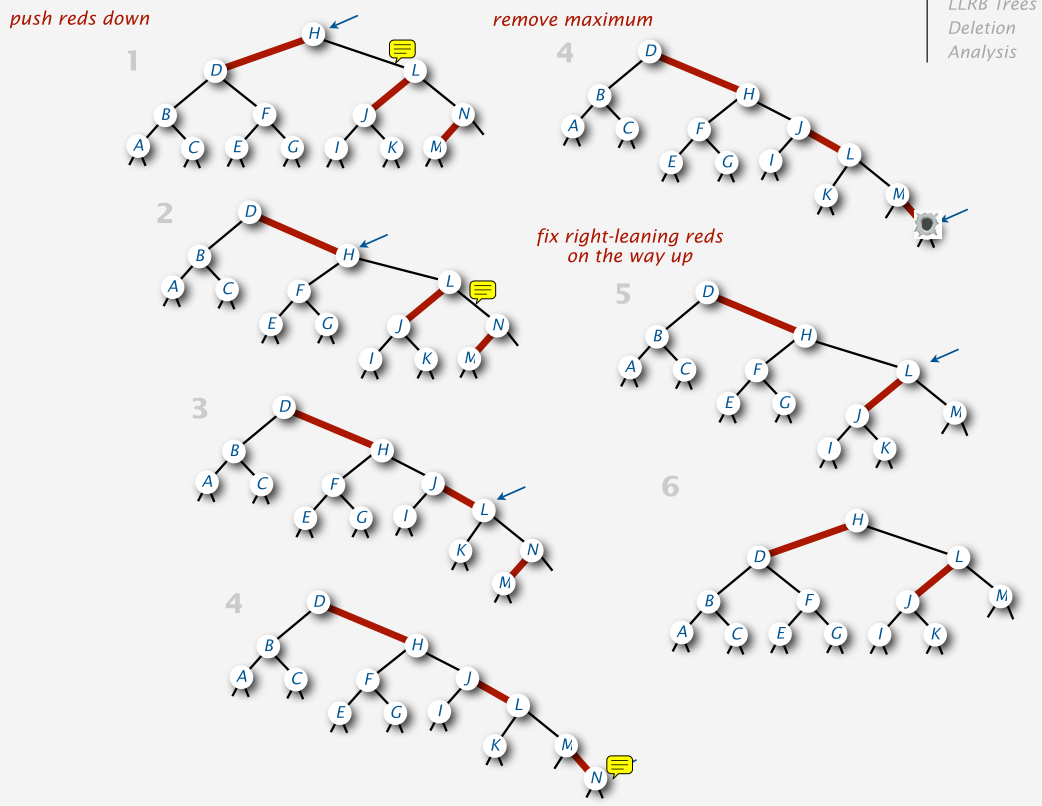### 删除普通的元素

1.我们需要注意：

• h和他的任意一个孩子必须是红色的节点。
• 如果是沿着路径向左，要确保他的左孩子有红节点（moveRedLeft）
• 如果是沿着路径向右，要确保他的右孩子有红节点（moveRedRight）
• 总是在最底部删除元素
• 修复节点

2.几种情况：

• 如果被删除的键<h.key
• 如果已经到达了树底且被删除的键==h.key
• 如果还没有，又有两种情况：

1.key == h.key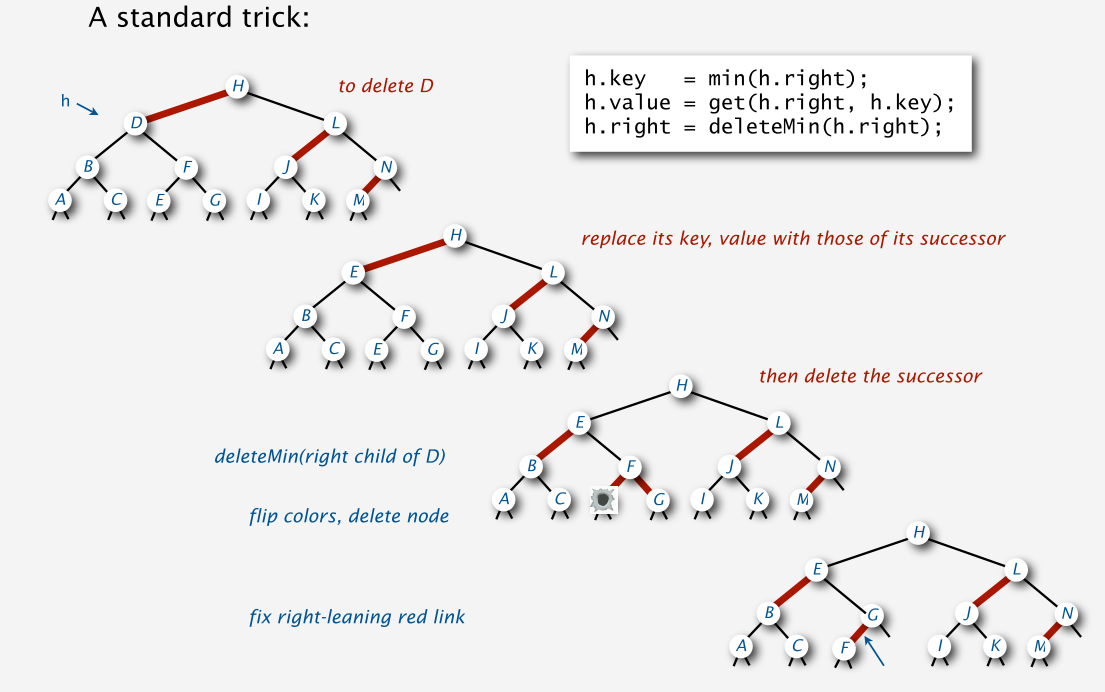2.key > h.key

C#实现：

//直接删除
private Node Delete(Node h, Key key)
{
if(key.CompareTo(h.key) < 0)
{
if (!IsRed(h.left) && !IsRed(h.left.left))
h = moveRedLeft(h);//左边还没有红节点，需要借一个
h.left = Delete(h.left, key);
}
else
{
//待删除的key>=h.key
//确保在右子树中能出现红色右孩子
if (IsRed(h.left))
h = rotateRight(h);
if (key.CompareTo(h.key) == 0 && (h.right == null))
return null; // 在树底找到要删除的键
if (!IsRed(h.right) && !IsRed(h.right.left))//确保删除的节点不为2节点，
h = moveRedRight(h);
if (key.CompareTo(h.key) == 0)
{
//h.key == key
//属于待删除的节点，那么使用代替删除的方式，从右子树中选择最小的节点，修改他的值。然后在右子树中删除这个最小的键
Node temp = Min(h.right);
h.key = temp.key;
h.val = temp.val;
h.right = DeleteMin(h.right);
}
else
{
//如果节点>h.key
h.right = Delete(h.right, key);
}
}
return Balance(h);
}
public override void Delete(Key key)
{
if(Contains(key))
{
if (!IsRed(root.left) && !IsRed(root.right))
root.color = RED;
root = Delete(root, key);
if (!IsEmpty())
root.color = BLACK;//头节点是红色的。因为删除一个节点可能会把头结点变红
}
}
private bool Contains(Node head, Key key)
{
{
if (cmp > 0)
else if (cmp < 0)
else
return true;
}
return false;
}
public override bool Contains(Key key)
{
return Contains(root, key);
}

# 参考&引用

1. （Wikipedia）红黑树
2. （CSDN）红黑树插入操作
3. （CSDN）红黑树的删除节点操作
4. Robert Sedgewick等，算法（第四版）[M]. 北京：人民邮电出版社，2012：269-292